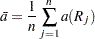### One-Way ANOVA Tests

PROC NPAR1WAY computes a one-way ANOVA test for each score type that you specify. Under the null hypothesis of no difference among class levels (samples), this test statistic has an asymptotic chi-square distribution with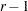degrees of freedom, whereis the number of class levels. For Wilcoxon scores, this test is known as the Kruskal-Wallis test.

Exact one-way ANOVA tests are also available for multisample data (where the data are classified into more than two levels). For two-sample data, exact simple linear rank tests are available. PROC NPAR1WAY computes exact tests for each score type that you specify in the EXACT statement. See the section Exact Tests for details.

PROC NPAR1WAY computes the one-way ANOVA test statistic as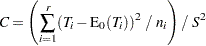where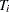is the total of scores for class level,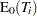is the expected total for levelunder the null hypothesis of no difference among levels,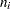is the number of observations in level, and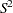is the sample variance of the scores. The total of scores for class levelis given by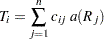where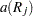is the score for observation, and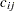indicates whether observationis in level. The expected total of scores for class levelunder the null hypothesis is equal to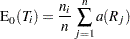The sample variance of the scores is computed as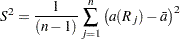whereis the average score,